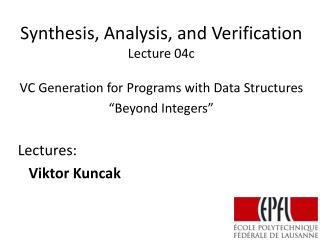DownloadDownload PresentationSynthesis, Analysis, and Verification Lecture 04c

# Synthesis, Analysis, and Verification Lecture 04c

Télécharger la présentation## Synthesis, Analysis, and Verification Lecture 04c

- - - - - - - - - - - - - - - - - - - - - - - - - - - E N D - - - - - - - - - - - - - - - - - - - - - - - - - - -
##### Presentation Transcript

1. Synthesis, Analysis, and VerificationLecture 04c VC Generation for Programs with Data Structures “Beyond Integers” Lectures: Viktor Kuncak

2. Verification-Condition Generation (VCG) • Steps in Verification • generate formulas implying program correctness • attempt to prove formulas • if formula is valid, program is correct • if formula has a counterexample, it indicates one of these: • error in the program • error in the property • error in auxiliary statements • Terminology • generated formulas: verification conditions • generation process: verification-condition generation • program that generates formulas: verification-condition generator (VCG)

3. VCG Explained Until Now Programs that Manipulate Integers Compute Formulas from Programs Formulas with Integer Variables and Operations Prover (Integer Constraint Solver)

4. VCG for Real Languages Programs that Manipulate Integers,Maps, Arrays, and Linked Data Structures Compute Formulas from Programs have more operations in expressions of x=E Formulas with Integer Variables and Operations, as well as variables and operations on functions Prover (Integer Constraint Solver) + provers for function symbols, mathematical arrays, term algebras, ...

5. Weakest Precondition Formula For set P, relation r P = wp(r,Q) means Let PF and QF have x as free variable(s) For formula QF , command c, PF = wp(c,QF) should imply {x | PF} = wp([[c]], {x|QF}) If formula for command c is F(x,x’) then PF is

6. Preconditions for Basic Commands assume(E) x=E havoc(x)

7. Key Next Step: Handling Arrays If we know how to handle one static array,we will easily generalize to heap, many arrays,and other memory data structures. Now our language has • integer variables: x:Int; j:Int (as before) • but also arrays: a : Array[Int], b : Array[Int]

8. Subtlety of Array Assignment Rule for wp of assignment of expression E to variable x, for postcondition P: wp(x=E , P) = Example: wp(x=y+1,x > 5) = wp of assignment to an array cell: wp(a[i]=y+1, a[i]>5) = wp(a[i]=y+1, a[i]>5 && a[j]>3) =

9. wp of a[i]=E Let P be any formula containing also a[j] expressions wp(a[i]=E, P) =

10. Arrays as Mathematical Functions Suppose we have expressions that manipulate functions. Array update operator on functions: f(x:=v) = g means: 1) g(x)=v, and 2) g(y)=f(y) for y != x. How to represent assignments? x = a[i]  x = a(i) a[i]=v 

11. Construct formulas recursively Guarded program given by tree Leaves: x=E, assume(P) assume(P)  x=E

12. Tree nodes (recursion) Non-deterministic choice [] Sequential composition ;

13. Generated Formula: Size and Structure How do generated formulas look like for loop-free code? ((c1 ; c2) [] (c3 ; c4)) ; c5 ( F1 & F2 | F3 & F4 ) & F5 can move existential quantifiers to top What is the size of the formula as function of code size?

14. Logic with Array Updates Variables denote: integers or arrays Operations on integers: +,-,*,/ Operations on arrays: a(i), a(i:=v) Comparison operators: ==, !=, <, > Operators on atomic formulas: &&, ||, ! (Combination of theory of integers and extensional theory of arrays.)

15. Example with Static Arrays if (a[i] > 0) { b[k]= b[k] + a[i]; i= i + 1; k = k + 1; } else { b[k] = b[k] + a[j]; j= j + 1; k = k – 1;}

16. Example with Static Arrays • formula • guarded commands: (assume(a(i) > 0); b= b(k:= b(k)+ a(i)); i= i + 1; k = k + 1;) [] (assume(a(i)<=0); b= b(k:= b(k)+ a(j)); j= j + 1; k = k – 1;)

17. Conditional Expressions y = (x > 0 ? x : (-x)) y = abs(x) a3 = a2(i:=v) && x = a3(j) Can we eliminate a3? We obtain

18. Eliminating Conditionals Formula u = (x > 0 ? x+1 : 2-x) becomes: Satisfiability of y > z + 2*(x > 0 ? x : (-x)) Becomes satisfiability of Satisfiability of disjunctions?

19. Logic with Conditional Expressions Variables denote: integers or arrays Operations on integers: +,-,*,/ Arrays access: a(i) Comparison operators: ==, !=, <, > Operators on atomic formulas: &&, ||, ! (Combination of theory of integers and theory of functions.)

20. Suppose we find values for integersWhen can we find functions? f(i) = x && f(j) = y i  0, j  1, x  5, y  7example f: f(i) = x && f(j) = y && i == j i  1, j  1, x  5, y  7example f: Note if we have f(f(i))=x 

21. Satisfiability of Constraints with Uninterpreted Functions If we have a model for integer values such that then we can extend it to a model of functions. How to ensure we only find models that satisfy constraints? (Ackermann encoding)

22. Now we can handle static arrays wp(x=E, P) =

23. Reference Fields class Node { Node next; } How to model ‘next’ field? y = x.next;  x.next = y; 